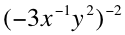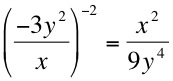# Math Help: Solving Negative Exponents

08/14/2015

## Algebra Help: How to Solve Exponents That Are Negative

An exponent represents the number of times you have to multiply a number by itself, but what if the exponent is negative or a fraction? Is it possible to multiply a number by itself a negative number of times or 1/2 times? While it can be a brain teaser, we will show you how to solve equations with negative and fraction exponents. It is actually quite simple, and we will show you a few examples.

### Negative Exponent Operation Definition

We recognize that you cannot multiply a number by itself a negative number of times, so what does 2^(-4) mean? A negative exponent is equal to the inverse of the same number with a positive exponent. 2^(-4)=1/2^4=1/16. Solving a negative exponent is that easy.

### Examples

Here are a few more examples for you to try at home. 3^-2=1/9 4^-1=1/4 2^-5=1/32 1/(2^(-5))=2^5=32

### Using Variables

Replacing your number with a variable keeps everything the same, but with a letter. Just flip your number if it is a negative exponent. Let's try some more complicated examples.

### Examples with Variables

Simplify the following equation:Because exponents work with multiplication, it does not matter which order you do them. You can either start with the -2 exponent outside then move inside, or vice versa. Let's start inside first.## For Tutoring in Kansas City, MO

If you are looking for more help with math, reading, writing, or test prep, check out the one-to-one tutoring programs at The Tutoring Center in Liberty-Kansas City. Call 816-781-0000 today and ask for our free diagnostic assessment.

### FREE CONSULTATION

Schedule your Free Diagnostic Assessment Today!
Learn more about
THE TUTORING CENTER
on the national website: